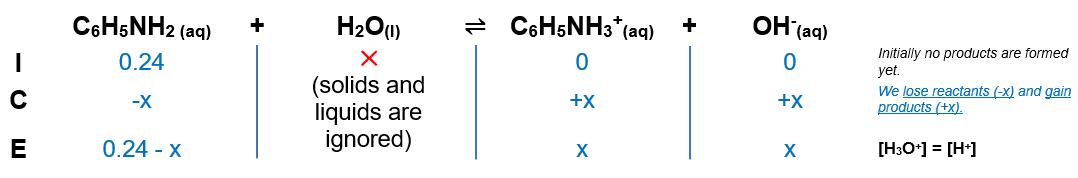# Problem: What is the pH of a 0.24 M solution of aniline (C6H5NH2, Kb = 3.8 × 10–10) at 25°C?A) 2.00B) 5.02C) 10.04D) 13.38E) 8.98

###### FREE Expert Solution

We’re being asked to calculate the pH of a 0.24 M aniline solution given the Kb of 3.8x10-10

In this case, we have to follow the steps:

Step 1. Setup the equilibrium and ICE chart

Step 2. Calculate x or OH-

Step 3. Calculate pH

Step 1. The formula for aniline is C6H5NH2 and its reaction with water is:

C6H5NH2 +  H2O  ⇌  C6H5NH3+    +  OH-

We need to write an ICE chart to determine the concentration of OH- which we will use to get the pOH, then the pH.We know that the equilibrium constant expression, Kb is:

88% (89 ratings)###### Problem Details

What is the pH of a 0.24 M solution of aniline (C6H5NH2, Kb = 3.8 × 10–10) at 25°C?

A) 2.00

B) 5.02

C) 10.04

D) 13.38

E) 8.98